# What Does Term Mean in Algebra?What is a term?

In algebra, term can be defined as the values on which the mathematical operations occur in an expression. A term can either be a constant or a variable or it can be both in an expression.

Let us take the expression 3a + 8. In this expression, 3a and 8 are terms. Another example is 5x + 7 where 5x and 7 are terms.

Expression: Term, Factor and Coefficient

An algebraic expression is a combination of constants and variables which are connected by the mathematical operators like +, -, *, and division. Constant can be defined as the numbers which have a fixed numerical value. Variables can be defined as the numbers which can express various numerical values. The value of an expression relies on the values of the variables of an expression.

Definition of Expression

The mathematical statements are expressed in algebraic expressions. For example, when it is said that c is doubled, then it is expressed as 2c. The expression 2 less than t is expressed as t-2. Thus, 2c and t-2 are algebraic expressions. An expression contains the terms, factors, and coefficient. Terms are derived when the numbers or the variables are added together. The factors are derived when the numbers or the variables are multiplied together. The coefficient is derived when the number is multiplied to the variable. In the expression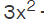+ 5x + 2,,5x and 2 are the terms, the variables are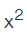and x, 2 is the constant and the coefficient ofis 3, and the coefficient of x is 5. Therefore, an expression is made up of terms, variables, factors, coefficients, and constants.

Term, Factors and Coefficient of Expression

Now let us understand the various parts of an expression with the help of an example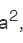– 6a + 9.

• the terms are6a and 9
• the variables areand a
• the constant is 9
• the factors are a, a are the factors of term 1, -6 and a are the factors of term 2, and 9 is the factor of term 3.
• 1 is the coefficient of-6 is the coefficient of a, and 9 is the coefficient of the constant term.
• The operators are – and +.

Terms

When many parts of an algebraic expression are separated by = or – sign, then they are called terms of the expression. The terms may appear to be as single numbers, variables, or the product of a number and variable. The polynomials are divided on the basis of the number of terms such as monomial, binomial, trinomial. The word polynomial is derived from the Greek word poly and nomial which means terms. The terms can be either like or unlike terms.

•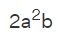is monomial because it has one term.
• 2x – 3 is binomial because it has two terms.
•+ 3x + 2 is trinomial because it has 3 terms.

Factors

Factors are a part of the product. 5y means 5 * y and y are multiplied together to make 5y. Therefore, both are the factors of the term 5y. When the term is expressed as a product of 2 or more variables or numbers, then it is called factorization. Any given algebraic expression might need to be factorize. For example,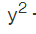+ y is factorized as y(y + 10), meaning that y and y + 1 are factors of+ y and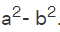= (a + b)(a – b), meaning (a+ b) and ( a- b) are the factors ofCoefficient

The coefficient is the number or a variable which is multiplied to another variable in the expression. Let us take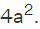Here, 4 is the numerical coefficient ofandis the literal coefficient of 4. Take another example of 12n. here 12 is the numerical coefficient of n. When no number is there, then it is understood that 1 is the coefficient. Let us take the expression+ b. Here, 1 is taken as the numerical coefficient ofand also b.

The terms which have the same literal coefficients are known as like terms, whereas the terms which have different literal coefficients are known as the unlike terms.

• Like terms are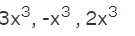• Unlike terms are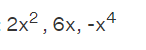Key points

• The mathematical statements which are expressed in words are shown using variables in algebra and they make the algebraic expression.
• An expression is made up of terms, variables, constants, factors, and coefficients. The mathematical operators + or – comes in between the terms.

For any queries related to above such topics on mathematics learn more on Algebraic Expressions.